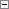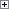Use this simple data type to store a single, 16-bit character as a value in the range 0 to 65535. You can convert this data type from a number to a character and vice versa. This means you can use mathematical operators on Char variables.Example

The following example assumes that you have a Char variable named C and a Text or Code variable named S.

You can assign a constant string of the length 1 to a Char variable, as shown in the first line of the following code example.

You can assign a single Char in a Text or Code variable to a Char variable, as shown in the second line of the following code example.

You can assign a numeric value to a Char variable, as shown in the third line of the following code example.

A Char variable represents Unicode characters in the same way as the .NET Framework Char structure. For more information about the .NET Framework Char structure, see Char Structure.Copy Code
C := 'A';
C := S;
C := 65;

You cannot assign a Char to a position greater than the position of the null terminator. For example, if the value of the Text variable MyText is 'abc', then the null terminator is at position 4 and the following assignment causes a run-time error to occur.Copy Code
MyText := 'e';